31% percent of surveyed students report that finals week is the most stressful time of the semester. However, the exams are rarely the last of a senior’s worries.

Understanding how much you need to score to get the desired grade for a course will help you see where you stand – and where you need to be.

This guide highlights all of the different methods to calculate your final grade.

## How to Calculate Weighted Final Grades

### Method #1: Manual Calculation

Before you use this method, you must note down three things: your current grade, the weight of the final exam, and the grade you want on the course.

Students use this method to determine what grade they need on their final to get a specific grade on the entire course.

For the sake of explanation, let’s assume your current grade is 86%, the final exam accounts for 40% of your grade, and you want a 90% grade on the course.

#### Step #1: Find Weight of Course

First, you must find the weight of your current grade. You can do this by subtracting the final exam’s weight from 100%.

Since the final exam weighs 40% of the total grade, the rest of the course will account for 60% of your total grade (100% – 40% = 60%).

#### Step #2: Determine Weighted Value of Current Grade

In step #1, we calculated the weight of the course, excluding the final exam. In this step, we must determine what the weighted value of the current grade is.

The current grade is 86%, and it accounts for 60% of the entire course’s grade. Therefore, we can find the weighted current grade by multiplying these two values.

86*60% = 86*0.6 = 51.6

Therefore, the Weighted Value of the Grade (WVG) in the example is 51.6.

#### Step #3: Subtract the WVG from the Desired Grade

Before you can find the grade you need on the final exam, you will need to subtract the WVG from your desired grade to move on to the final step.

In our example, the WVG is 51.6, and the desired grade is 90%.

90-51.6 = 38.4

#### Step #4: Find the Required Final Exam Grade

To find the score you need on the final exam to get the grade you want, you must divide the number you found in step #3 with the weight of the final in decimal.

From our example:

38.4/0.4 = 96

Therefore, to get a 90% on the course, you must score a 96 on the final exam.

Another way to find the grade you will need to score to get a specific grade on the course is to use spreadsheets.

#### Step #1: Open Up a Spreadsheet

When you have a spreadsheet open in front of you, you must label columns systematically to help you find the required final exam grade.

Label the first column “activity,” second column “score,” third column “maximum possible score,” fourth column “weight,” and the fifth column “weighted scores.”

After you label the columns, fill up the cells to match the course’s details. Your spreadsheet should look something like this: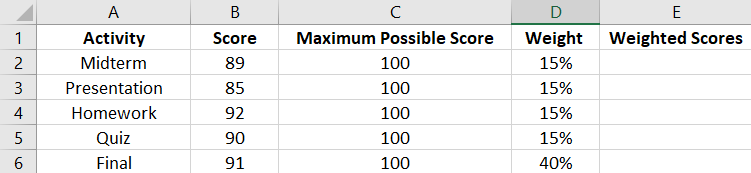#### Step #2: Find Weighted Scores

Before you can determine the course’s weighted grade, you will need to calculate the weighted scores of every individual activity.

Doing this is as simple as multiplying your score for every activity with its weight.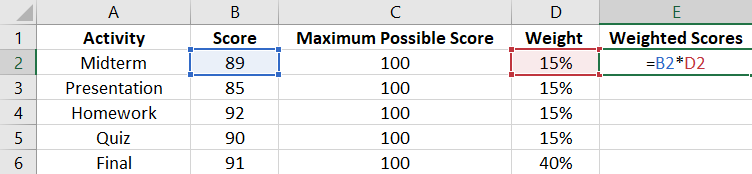#### Step #3: Add Up Weighted Scores

After finding the weighted scores of every activity, adding them up will give you the final grade.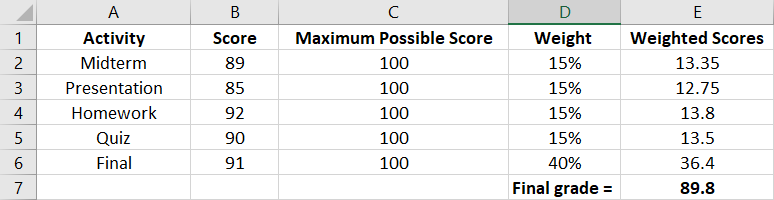Note: If you want to find the weighted average of your grades, divide the sum of the weighted scores by the sum of their weights. Alternatively, you could use a GPA calculator.

### Method #3: Using the Meta-Calculator Final Grade Calculator

You can enter all of your grades and their respective weights into Meta-Calculator. Then, calculating the final grades is as simple as hitting the “Calculate” button.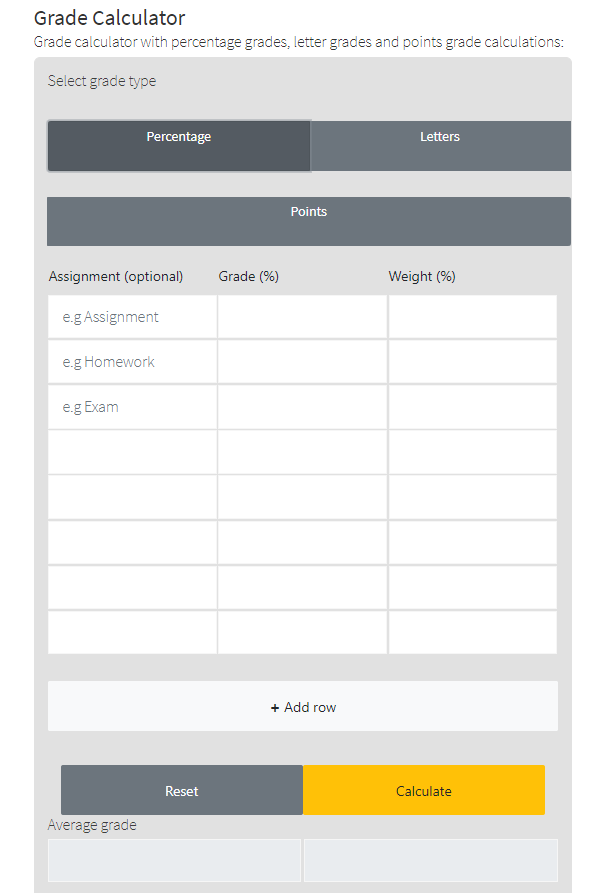On the other hand, if you want to calculate the score you need on your final to meet a specific target, you can use Meta-Calculator’s final grade calculator.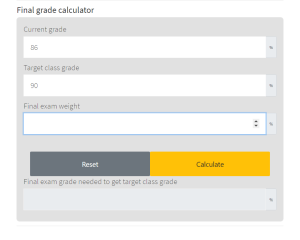All you have to do is enter your current grade, the target grade, and the final exam’s weight into the appropriate boxes.

When you hit the “Calculate” button, the grade you need on the final will appear in the box below.